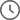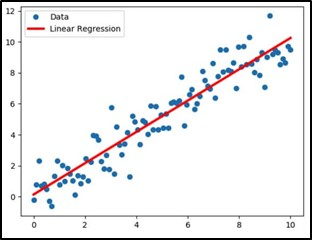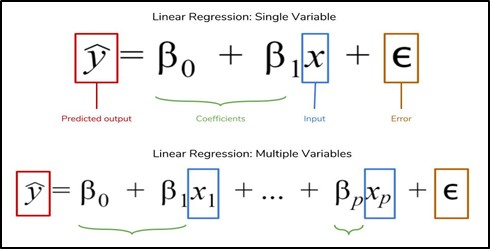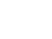AI/ML, Cloud Computing4 Mins Read

# Machine Learning Algorithm : Linear Regression

•• By Vinay Lanjewar
•March 13, 2023
 Voiced by Amazon Polly

## Introduction

Linear regression is a widely used statistical method for modeling the relationship between a dependent variable and one or more independent variables. It is a simple yet powerful tool that can help researchers and analysts understand the relationship between variables, make predictions, and test hypotheses. Linear regression is used in various fields, including economics, finance, social sciences, engineering, biology, etc. It is often used for tasks such as predicting sales, forecasting economic trends, analyzing survey data, and modeling the impact of interventions or treatments.

## Linear Regression

Linear regression is the basic supervised machine learning algorithm, with the help of which we will predict the relation between the dependent variable and independent variable, independent variable can also be known as features.

Considering the relation between the independent and dependent variable as how much the dependent variable will increase when there is an increase of one unit in the independent variable keeping all the independent variables constant.  From image 1, blue points are data, and red is the regression line.### Making IT Networks Enterprise-ready – Cloud Management Services

• Accelerated cloud migration
• End-to-end view of the cloud environment
Get Started

## Linear Regression Equation

From the image, linear regression for single and multiple variables is used.

Here,

y = Dependent or predicted variable

β = Coefficients

x = Independent variables

e = Random errorImage: Linear regression equation

With the help of the actual and predicted variables, we are going to calculate error with the help of various evaluation matrices such as MSE (Mean squared error), RMSE (Root mean squared error), MAE (Mean absolute error), and accuracy we will refer to R2 score (R squared score).

## Assumptions of Linear Regression

There are some assumptions of the linear regression, such as

• The dependent variable and independent variable should be almost linear.
• The mean of residual should be equal to “zero”.
• There should be homoscedasticity or equal variance in the regression model.
• There should not be multi-collinearity between independent variables.

• Linear regression is simple to implement.
• It is easy to use when we know the relation between data sets.
• It works well, irrespective of the data set.

## Limitations of Linear Regression

• Linearity assumption: Linear regression assumes the relationship between the dependent and independent variables is linear. This means that the effect of each independent variable on the dependent variable is constant across the range of values of that variable. However, this assumption may not hold in many real-world situations, and the relationship may be more complex.
• Outliers: Linear regression is sensitive to outliers, which are extreme values that deviate significantly from the other data points. Outliers can greatly impact the slope and intercept of the regression line, leading to inaccurate predictions. Therefore, it is important to identify and handle outliers before fitting a linear regression model.
• Multicollinearity: Linear regression assumes that the independent variables are not highly correlated. When two or more independent variables are highly correlated, it becomes difficult to determine the unique contribution of each variable to the dependent variable. Multicollinearity can also lead to unstable estimates of the regression coefficients and poor model performance.
• Heteroscedasticity: Linear regression assumes that the variance of the errors is constant across all levels of the independent variables. However, in some situations, the variance of the errors may increase or decrease as the values of the independent variables change. This is known as heteroscedasticity, which can lead to biased and inefficient estimates of the regression coefficients.
• Non-normality: Linear regression assumes that the errors are normally distributed with a mean of zero and constant variance. However, the errors may not follow a normal distribution in many real-world situations. Non-normality can lead to biased estimates of the regression coefficients and incorrect hypothesis testing.
• Overfitting: Linear regression can suffer from overfitting, which occurs when the model is too complex and captures noise in the data rather than the underlying relationship between the variables. Overfitting can lead to poor model performance on new data.

## Regularization technique

To overcome the disadvantages of linear regression, we use two regularization techniques: Ridge regression (L2) and Lasso regression (L1). Ridge regression shrinks coefficients but rarely takes the coefficient to “zero”. Lasso regression shrines the coefficient to “zero” removing features from the dataset.

Which regularization technique to use?

For accuracy purposes, it is better to use both Ridge and Lasso. Also, we can use a combination of these two as “Elastic Net” for the performance. Ridge performs better than Lasso.

## Conclusion

Linear regression is a supervised machine learning algorithm, easy to implement on the dataset irrespective of the size of the dataset.

It assumes a linear relation between the Independent and dependent variable, which is impossible in real-world problems.

Various evaluation matrices are used for evaluation, such as MSE (Mean Squared Error), RMSE (Root Mean Squared Error), and R2 score (R Squared score).

Linear regression generally underfits the data that it performs well on the training set with high accuracy and less accuracy on the test set.

### Get your new hires billable within 1-60 days. Experience our Capability Development Framework today.

• Cloud Training
• Customized Training
• Experiential Learning

CloudThat is also the official AWS (Amazon Web Services) Advanced Consulting Partner and Training partner and Microsoft gold partner, helping people develop knowledge of the cloud and help their businesses aim for higher goals using best in industry cloud computing practices and expertise. We are on a mission to build a robust cloud computing ecosystem by disseminating knowledge on technological intricacies within the cloud space. Our blogs, webinars, case studies, and white papers enable all the stakeholders in the cloud computing sphere.

Drop a query if you have any questions regarding Linear Regression and I will get back to you quickly.

To get started, go through our Consultancy page and Managed Services Package that is CloudThat’s offerings.

## FAQs

1. What is the difference between simple and multiple linear regression?

ANS: – Simple linear regression models the relationship between a dependent variable and a single independent variable. Multiple linear regression involves modeling the relationship between a dependent variable and multiple independent variables.

2. How do you handle outliers in linear regression?

ANS: – Outliers can be handled by removing them from the dataset or transforming them using methods such as log transformations. Another approach is to use robust regression methods less sensitive to outliers.

3. What is the difference between a dependent and independent variable in linear regression?

ANS: – The dependent variable is predicted or modeled in linear regression, whereas the independent variable is used to predict or model the relationship.

4. Can linear regression be used for categorical variables?

ANS: – Linear regression can be used for categorical variables by converting them into dummy variables or using other techniques, such as logistic regression.#### WRITTEN BYVinay LanjewarClick to Comment